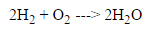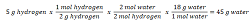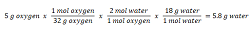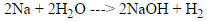# Limiting Reactant Practice Problems

Instructor: Laura Foist

Laura has a Masters of Science in Food Science and Human Nutrition and has taught college Science.

In this lesson, we're going to practice finding the limiting reactant. First, we'll review how to find the limiting reactant, then we'll solve two practice problems.

## Limiting Reactants

In everyday life, finding the limiting reactant isn't that difficult, as long as you know what to look for. For example, if you have three storage containers but just two lids, you can only put together two complete sets of containers and lids. In this instance, the three lids are the limiting reactants, while the lidless container is the excess reactant. The limiting reactant is the amount of a chemical substance that is consumed, as well as limits how much product forms, during a chemical reaction.

In chemistry, calculating the limiting reactant can be a little confusing because we need to change from grams (g) to moles (mol) in order to have a comparable unit. To perform this calculation, we need the following three pieces of information:

1) The balanced equation

2) The molecular weight of reactants and products

3) The amount of reactants used

Once we have this information, we can complete the following three steps:

Step 1) Figure out how many moles of reactant we have.

Step 2) Multiply the moles of reactant by the ratio of product to reactant.

Step 3) Change moles of product into grams of product.

## Practice Problem One

In this problem, we'll be creating water from hydrogen and oxygen using the information below.

1) The balanced equation2) The molecular weight of each element or compound: hydrogen (2 g/mol), oxygen (13 g/mol) and water (18 g/mol)

3) The 5 grams of hydrogen and 5 grams of oxygen used

Step 1: Convert the 5 grams of hydrogen to moles; so 5/2 = 2.5 moles of hydrogen.

Step 2: Multiply the ratio of product to reactant, or 2:2; so 2.5(2/2) = 2.5 moles of water.

Step 3: Convert from moles to grams of water; so 2.5(18) = 45 grams of water.

Now we do the same thing with the oxygen:

Step 1: Convert the 13 grams of oxygen to moles; so 5/32 = 0.16 moles of oxygen.

Step 2: Multiply the ratio of product to reactant, or 2:1; so 0.16(2/1) = 0.32 moles of water.

Step 3: Convert from moles to grams of water; so 0.32(18) = 5.8 grams of water.

Since the 5 grams of oxygen will only produce 5.8 grams of water, while the 5 grams of hydrogen could produce 45 grams of water, the oxygen is our limiting reactant: 5.8 < 45.

One of the best ways to check your work is to set up a stoichiometry equation, or a mass-mass equation, so that you can make sure that all of the units properly cancel out. Here is the stoichiometric equation for hydrogen:And here is the stoichiometric equation for oxygen. Do you see how the grams and moles of hydrogen cancel each other out?You can also check each individual fraction. Does each fraction equal 1?

Step 1: One mole of hydrogen = 2 grams of hydrogen; so 1 mole hydrogen / 2 grams hydrogen = 1.

Step 2: Two moles of hydrogen = 2 moles of water; so 2 moles of water/2 moles hydrogen = 1.

Step 3: One mole of water = 18 grams of water; so 18 grams water/1 mole water = 1.

In this way, we can check our work. If there is more than one product, we can also check our work by performing the same calculations with the other product. If we come out with the same limiting reactant, then we did it correctly.

## Practice Problem Two

Now we are going to create sodium hydroxide and hydrogen from sodium and water using the information below.

1) The balanced equation2) The molecular weight of each element or compound: sodium (23 g/mol), water (18 g/mol), sodium hydroxide (40 g/mol) and hydrogen (2 g/mol)

3) The 10 grams of sodium and 15 grams of water used

Let's start with sodium. And we will use hydrogen as the product, but you can use either product.

Step 1: Convert the 23 grams of sodium to moles; so 10/23 = 0.4 moles of hydrogen.

Step 2: Multiply the ratio of product to reactant, or 1:2; so 0.4(1/2) = 0.2 moles of hydrogen.

Step 3: Convert from moles to grams of hydrogen; so 0.2(2) = 0.4 grams of hydrogen.

Now we do the same thing with the water:

Step 1: Convert the 18 grams of water to moles; so 15/18 = 0.8 of water.

To unlock this lesson you must be a Study.com Member.

### Register to view this lesson

Are you a student or a teacher?